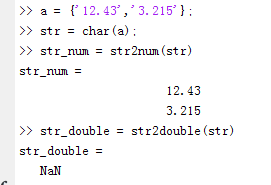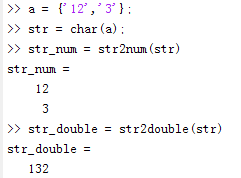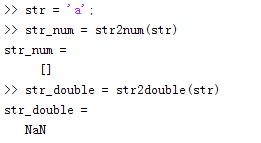• double函数：只是简单地把一个变量类型转换成double类型，而数值本身大小不变。 im2double函数：将输入类型皈依化到0到一之间，如果输入本身是double类型，不进行归一化。 matgray函数：是将图像矩阵进行归一化。 ...
double函数：只是简单地把一个变量类型转换成double类型，而数值本身大小不变。
im2double函数：将输入类型皈依化到0到一之间，如果输入本身是double类型，不进行归一化。
matgray函数：是将图像矩阵进行归一化。

为什么要进行归一化处理？
matlab里图像数据有时候必须是浮点型才能处理，而图像数据本身是0-255的UNIT型数据所以需要归一化，转换到0-1之间。
在实际的对图像处理过程中，由于我们读入图像是unit8型，而在MATLAB的矩阵运算中要求所有的运算变量为double型（双精度型）。因此通常使用im2double函数将图像数据转换成双精度型数据。

关于一幅图像矩阵经过double函数转换后，用imshow函数显示图像发现图像全白。
因为在matlab中使用imshow()函数来显示图像，而此时的图像矩阵可能经过了某种运算。在matlab中，为了保证精度，经过了运算的图像矩阵I其数据类型会从unit8型变成double型。如果直接运行imshow(I)，我们会发现显示的是一个白色的图像。这是因为imshow()显示图像时对double型是认为在0~1范围内，即大于1时都是显示为白色，而imshow显示uint8型时是0~255范围。而经过运算的范围在0-255之间的double型数据就被不正常得显示为白色图像了。


展开全文• str2num 函数与 str2double 函数的相同点与不同点 1. 相同点：当str为一个含数字的字符串时，str2num 函数与 str2double 函数一样。 如： 2. 不同点：当str为多个字符串构成的数组时，str2num 函数与 str2...
str2num 函数与 str2double 函数的相同点与不同点

1. 相同点： 当str为一个含数字的字符串时， str2num 函数与 str2double 函数一样。

如：2. 不同点：当str为多个字符串构成的数组时， str2num 函数与 str2double 函数有区别。

如：不同点：当str为非数字字符时，str2num 函数与 str2double 函数有区别。

如：展开全文str2num
• ## Matlab cellfun函数

万次阅读 2017-10-30 16:11:04
Matlab中cellfun函数
Matlab中有一个数组类型,叫做Cell,Cell是一种广义数组,他的每个元素称之为一个单元,每个单元可以是任意类型数组,比如数值数组,字符串,结构体或者一个子Cell.因此每个单元可以有不同的大小.

cellfun是将一个函数运用到Cell数组中的所有元素

语法:

[A1,…,Am] = cellfun(func,C1,…,Cn)
[A1,…,Am] = cellfun(func,C1,…,Cn,Name,Value)
[A1,…,Am] = cellfun(func,C1,…,Cn)调用函数句柄func指定的函数,并传递Cell数组中的元素C1,…,Cn,n是传递到函数的参数个数,输出数组A1,…,Am,m为func函数输出的数量.第i次迭代对应于语法为[A1(i),…,Am(i)] = func(C1{i},…,Cn{i})。cellfun函数不能指定顺序来执行func函数。

[A1,…,Am] = cellfun(func,C1,…,Cn,Name,Value)调用函数func,附加选项通过一个或多个”Name,Value”键值对参数指定,Name可以取值”UniformOutput”或者”ErrorHandler”.

键值对参数

‘UniformOutput’:逻辑值如下 true 表示对于所有输入，函数func的每一个输出都是一个标量单元数组，标量结构，或者一个总是相同类型和大小的标量值。cellfun函数将输出组合为A1,…Am的数组。每个输出数组都与单独的函数输出类型相同。
false要求cellfun函数将输出组合成单元数组A1，…，Am。函数func的输出可以是任何大小或类型。
‘ErrorHandler’ 一个函数句柄,捕获所有MATLAB执行此函数遇到的错误,定义这个函数来重新抛出错误或者返回一个有效的输出.
Examples
计算Cell数组中元素的平均值.

    C = {1:10, [2; 4; 6], []};
averages = cellfun(@mean, C)
% 返回结果
averages =    5.5000    4.0000       NaN
[nrows, ncols] = cellfun(@size, C)
% 返回结果
nrows =
1     3     0
ncols =
10     1     0

创建一个包含字符向量的单元数组，并将其缩写为前三个字符。由于输出字符矢量为非标量，因此将UniformOutput设置为false。

days = {'Monday', 'Tuesday', 'Wednesday', 'Thursday', 'Friday'};
abbrev = cellfun(@(x) x(1:3), days, 'UniformOutput', false)
%   abbrev =     'Mon'    'Tue'    'Wed'    'Thu'    'Fri'

语法 @(x)创建了一个匿名函数.

计算两个单元阵列C和D之间的协方差，因为协方差输出是非标量，将UniformOutput设为false。

c1 = rand(5,1);  c2 = rand(10,1);  c3 = rand(15,1);
d1 = rand(5,1);  d2 = rand(10,1);  d3 = rand(15,1);
C = {c1, c2, c3};
D = {d1, d2, d3};

covCD = cellfun(@cov, C, D, 'UniformOutput', false)
% covCD =     [2x2 double]    [2x2 double]    [2x2 double]


定义并调用自定义错误处理函数。

function result = errorfun(S, varargin)
warning(S.identifier, S.message);
result = NaN;
end

A = {rand(3)};
B = {rand(5)};
AgtB = cellfun(@(x,y) x > y, A, B, 'ErrorHandler', @errorfun, ...
'UniformOutput', false)
展开全文• ## matlab常用函数

千次阅读 2019-09-07 16:33:57
 一、软件操作函数
1）命令窗口函数：
clc：清空命令窗口，使用向上箭头翻看命令。
format：设置数据输出格式。format（'command'）
disp:在commandwindow中显示字符和矩阵。
disp(x)---x为矩阵
disp('x')---x为字符
disp(['x' a 'y'])---打印字符合集
clear：从工作空间中清空变量。clear, clear name,clear global name, clear –regexpexpr1 expr2
clear:清空工作空间的所有变量
clear name---clear('name'):清空以name命名的m文件，mex文件或变量名
clear globe name:完全清空以name命名的全局变量，清空后该全局变量不能被函数调用。

2)帮助函数
demo：通过help浏览器访问工具箱的演示文件。---demo'MATLAB'
doc:在help浏览器中打开相应帮助页面。
help：在MATLAB命令窗口中显示MATLAB的m函数，mdl文件，工具箱等的帮助信息。

二、文件编程函数
1)文件目录操作函数
dir:显示当前工作空间目录文件   dir; dirname;
files=dir('directory'):返回指定目录中的文件信息到结构体file中，结构体file中包含的信息为：name,data,bytes,isdir,datenum

2)文件编辑调试函数
dbstep:从当前断点中执行一行或多行代码  dbstep; dbstep nlines;dbstep in
dbstop:设置断点    dbstop inmfile  ; dbstop in mfile at lineno
debug:列举出m文件的调试函数
mlint:检查m文件可能出现的问题，并报告结果---mlint('m-filename')
profile:调试优化m文件代码---界面Desktop--Profiler

3)文件脚本函数
function:声明函数   function [out1, out2, ...]=funname(in1,in2,...)：定义一个名为funname的函数，该函数输入参数为in1、in2、……输出参数为out1、out2、……
input:请求用户输入   user_entry=input('prompt') ：在屏幕上显示prompt作为提示，等待键盘输入，并返回用户输入的值。user_entry=input('prompt','s')：将键入的字符返回为文本变量而非变量名或数值。

4）语句控制函数
break:终止执行for循环或者while循环, break语句结束循环，把语句控制返回给循环结束后的语句。
continue:传递控制给下一个for或while循环
case:条件为真时执行代码,case是switch语法中的允许有条件执行语句的一部分。case语句块由case函数，case表达式和其后的一条或多条语句构成。
switch:有条件执行的代码，在基本语法中，当switch_expr ==case_expr时执行相关语句，如果没有实例表达式与switch表达式相匹配，则语句控制传递给otherwise语句块。
实例：

 

method='bigger';

switch method

case'bigger'

a=3;

case'middle'

a=2;

case'small'

a=1;

otherwise

a=0;

end

If:如果条件为真，则执行相关语句   if expression, statements, end
elseif:---if expression1,statement1,elseifexpresstion,statement2,end
else:如果条件为假时执行语句
end:终止代码块，或为数组的最后一位数字索引, end用来终止for、while、switch、try和if语句---end函数也作为数组的最后一个索引，如X(3:end)和X(1,1:2:end-1)。可以用end增加数组，如X存在的情况下使用X(end+1)=5。
error:显示信息并终止函数---error('说明信息')
for：反复运算指定代码块  forvariable=initval:endval, statements, end
while:当条件为真时重复执行语句---whileexpression,statement,end
return:从调用函数中返回

5)内存控制函数
global:声明全局变量   初始化为一个空矩阵
isglobal:判断一个变量是否是全局变量
persistent:定义静态全局常量

第二篇：数据类型函数
三、基本数据类型函数
1）数值函数
double：转换为双精度浮点数
single:转换为单精度浮点数
typecast：在不改变数据大小情况下转换数据类型  Y=typecast(X,type)
type可选范围:'uint8'、'int8'、'uint16'、'int16'、'uint32'、'int32'、'uint64'、'int64'、'single'、'double'
arrayfun:把函数应用到数组中的每个元素 A=arrayfun(fun, S)应用fun函数到数组S中的每个元素，将结果返回给数组A。
A=arrayfun(fun, S, T, …)
实例：

x(1).f1=2;x(2).f1=3;x(1).f2=3;x(2).f2=3;result=arrayfun(@(x)isequal(x.f1,x.f2),x)

cast:转变数值数据类型  B=cast(A,newclass)
实例：

 

a=int16(2);

b=cast(a,'char');

cate=class(b)

cat:连接数组   C=cat(dim, A,B)--cat(2,A,B)与[A,B]相同，cat(1,A,B)与[A;B]相同
class:创建对象或者返回对象类型   obj=class(s,'class_name')
find：寻找非零元素的索引和值
ind=find(X)；X可以是逻辑式，返回非零元素的索引
ind=find(X, k, 'first') 返回数组X中所有非零元素的前k个元素。
ind=find(X, k, 'last')  返回数组X中所有非零元素的后k个元素。
[row,col,v]=find(X,...)返回列向量，行向量，v表示非零值
isequal ：判断两个对象是否相等 比较结构体是否相等时，结构体中子域的顺序不重要  NaNs在此函数中认识不相等---isequal(A,B,...)相同返回1，不同返回0
isequalwithequalnans:把NaN视为相等的情况下判断两个数组是否相等

2）字符串转化数值函数
base2dec :把指定进制的数字字符串转换为十进制数  d=base2dec('strn', base)
bin2dec :把二进制数字字符串转换为十进制数  bin2dec(binarystr)
hex2dec :把十六进制数字字符串转换为十进制数  d=hex2dec('hex_value')
hex2num :把十六进制数字字符串转换为双精度浮点数 n=hex2num(S)
str2double :把字符串转换为双精度浮点数
str2num :把字符串转换为数字

3）数值转化为字符串函数
dec2base:把十进制数转换为指定基下的数值字符串 str=dec2base(d, base)
dec2bin :把十进制数转换为二进制数值字符串
dec2hex :把十进制数转换为十六进制的数值字符串
int2str :把整数转换为字符串
mat2str :把矩阵转换为字符串
num2str :把数字转换为字符串

四、高级数据类型函数
1）结构体函数
cell2struct :把元胞数组转换成结构体数组 s=cell2struct(c, fields,dim) fields指结构体数组的域名，fields可以说字符数组或者字符串元胞数组。
size(c,dim)==length(fields)%如果fields是元胞数组 size
(c,dim)==size(fields,1)%如果fields是字符数组
实例：

 

c={'Tom','math',80;'Jane','math',70}

feilds={'name','subject','grade'};

s=cell2struct(c,fields,2)

struct:创建结构体数组 s=struct('field1', values1, 'field2',values2, ...)
struct2cell :把结构体数组转换为元胞数组
structfun :把函数应用于结构体中的每一个子域 A=structfun(fun, s)

2)元胞数组函数
cell ：创建元胞数组    c=cell(n)c=cell(m,n)
celldisp ：显示元胞数组的内容
cellfun ：把函数应用于元胞数组中的每个元素  A=cellfun(fun, C, D,...)
cellplot ：以图形形式显示元胞数组的结构
实例：

 

c{1,1}='2-by-2';c{1,2}='eigenvalues ofeye(2)';

c{2,1}=eye(2);c{2,2}=eig(eye(2));

cellplot(c)

cellstr ：根据字符串数组创建字符串元胞数组
mat2cell ：把矩阵分割为元胞数组  c=mat2cell(x,m, n)
num2cell ：把数值数组转变为元胞数组   C=num2cell(A)

3)字符串函数
func2str ：根据函数句柄构建函数名称字符串  s=func2str(fhandle)
char ：把整数数组转化为字符串  S=char(X)
eval ：执行包含MATLAB表达式的字符串  eval(expression)
findstr ：在长字符串中寻找匹配的短字符串,返回字符串索引
sprintf ：把一定格式把数据写入字符串   [s, errmsg]=sprintf(format,A, ...)
strcat ：水平连接字符串   t=strcat(s1,s2, s3, ...)
strcmp,strcmpi ：比较字符串---strcmp('str1','str2')相同返回1，不同返回0
strings函数：连接字符串name=['abc' 'def' 'ghj']
strrep :发现和替换子字符串   str=strrep(str1, str2, str3) ---将str1内的所有子字符串str2替换为str3
strtrim :删除字符串开头和结尾的空字符
blanks :创建空格字符串数组
isletter :判断字符串中字符是否为英文字母
isspace :判断字符串中字符是否为空格
isstrprop :判断字符串中是否含有指定类别的字符tf=isspace('str')
dblank :删除字符串末尾空格

第三篇：矩阵函数
五、数组和矩阵函数
1）数组基本函数
display：显示字符或者数组
isempty :判断数组是否为空，空返回1，不空返回0
isequal :判断数组是否相同 （认为NaN不同）
isequalwithequalnans:判断数组是否相同，把NaN看成相同的数
isfinite :判断数组元素是否为有限数
isfloat :判断数组元素是否为浮点数
isinf :判断数组元素是否为无限数
isinteger :判断元素是否为整数
islogical ：判断元素是否为逻辑变量
isnan ：判断元素是否为NaN
isnumeric ：判断数组元素是否为数值
isscalar ：判断输入是不是离散量
issparse ：判断矩阵是否为稀疏矩阵
isvector ：判断输入是否为向量
length ：计算向量的长度
max ：找出向量中的最大元素
C=max(A) A为一个向量，返回向量最大值，矩阵A，返回每列向量最大值
C=max(A,B) A、B维数一样，返回对应位置最大元素
max(A,[],dim)
[C,I]=max(A)找出最大值和索引
min ：找出向量中的最小元素
ndims ：计算矩阵的维数   ndims同length(size(x)) 一致
numel：计算数组中元素的个数或者下标数组表达式的个数
size ：计算数组维数大小  d=size(X) [m,n]=size(X)m行n列 m=size(X,dim)

2）数组操作函数
bsxfun：两个数组间元素逐个计算  C=bsxfun(fun,A,B)
@plus(加)@minus（减）@times(数组乘)@rdivide（左除）@ldivide（右除）@power（幂）@max@min@rem（整除）@mod（模除）
dot :向量点乘 C=dot(A,B)

3）基础矩阵函数
create :生成随机数流   [s1,s2,…]=RandStream.create('gentype','NumStreams',n)
eye :生成单位矩阵 Y=eye([m,n])对角线1，其他0
ones :生成元素为1的数组  Y=ones(n) Y=ones(m,n)
rand :生成均匀分布的伪随机数矩阵 r=rand(n) rand(m,n,p,…)
randi :生成均匀分布的伪随机整数矩阵 randi(imax) r=randi(imax,n)
r=randi(imax,m,n,p,…) r=randi([imin,imax],…) 例：r=randi([5,10],[2,3])
randn :生成[-1,1]之间随机分布数矩阵 r=randn(n) randn(m,n)randn(m,n,p,…)
randstream :生成随机数据流
set (RandStream) :设置随机数据流属性set(S,'PropertyName',Value)
set(S,'Property')

4)矩阵处理函数
reshape ：改变矩阵维数   B=reshape(A,m,n)
sort :把数组元素按升序或降序排列
B=sort(A,dim) B=sort(...,mode) [B,IX]=sort(A,2)返回B元素在A中的位置的索引
sortrows :矩阵行按照升序排列

5）矩阵分析函数
det :求方阵行列式值
norm :计算向量或者矩阵范数   n=norm(A) n=norm(A,p)
normest :返回矩阵2-范数估计值
null :计算矩阵零空间
rank :返回矩阵的秩
subspace :返回两个子空间夹角
trace :返回矩阵的迹对角元素之和

6）矩阵特征值函数
eig :计算矩阵特征值和特征向量
condeig :计算特征值对应的条件数
eigs :计算矩阵的最大特征值和特征向量
gsvd :矩阵广义奇异值分解
svd :矩阵奇异值分解

第四篇 数学函数
六、基本数学函数
1）基本运算符
+：加法运算符
-：减法运算符
*：矩阵乘法
.*：数组乘法
/：斜杠或者矩阵右除   B/A等于公式B*inv(A)
./：数组右除    A./B等于A(i,j)/B(i,j)
\：反斜杠或者矩阵左除    A\B等于inv(A)*B
.\：数组左除     A.\B等于B(i,j)/A(i,j)
^：矩阵幂计算
.^：数组幂计算    A.^B等于A(i,j)的B(i,j)次幂
’：矩阵转置
[]：表示空矩阵，A(m,:)删除A中的一行，A(:,n)删除A中的一列
{}：元胞组赋值
关系操作符：< <= > >= == ~ =
逻辑操作符 : 逻辑：&&  ||  数组：&  |  ~

2）数据操作函数
sort ：数组元素按升序或降序排列  B=sort(A) B=sort(A,dim) B=sort(...,mode)[B,IX]=sort(A,...)
sortrows ：把矩阵中每行按照升序排列   B=sortrows(A) B=sortrows(A,column)
sum ：求数列元素的和   B=sum(A) A是向量，向量求和，A是矩阵就没列求和 B=sum(A,dim)

3）统计分析函数
brush:交互式标记、删除、修改以及保存图片中的观测量位   data brush
corrcoef ：计算相关性系数  R=corrcoef(X)
cov ：返回协方差矩阵  cov(x)
max ：求数组中的最大元素     C=max(A) C=max(A,B) C=max(A,[],dim)
mean ：求数组的平均数或者均值  M=mean(A) M=mean(A,dim)
median ：返回数组的中间值  M=median(A) M=median(A,dim)
mode ：求数组中出现频率最多的值    M=mode(X) M=mode(X, dim)
[M,F]=mode(X, ...)
std ：计算标准差 s=std(X)
var ：计算方差  V=var(X)

4）三角函数
acos ：以弧度的形式返回反余弦值
实例：

 

x=-1:.05:1;

plot(x,acos(x));grid on

acosd ：以角度的形式返回反余弦值
acosh ：以弧度形式返回反双曲余弦值
acot ：以弧度的形式返回反余切值
acotd ：以角度的形式返回反余切值
acoth ：以弧度的形式返回反双曲余切值
acsc :以弧度的形式返回反余割值
acscd :以角度的形式返回反正割值
acsch :以弧度的形式反双曲余割值
asec :以弧度的形式返回反正割值
asecd :以角度的形式返回反正割值
asech :以弧度的形式返回反双曲正割值
asin :以弧度的形式返回反正弦值
asind :以角度形式返回反正弦值
asinh :以弧度的形式返回反双曲正弦值
atan :以弧度的形式返回反正切值
atand :以角度的形式返回反正切值
atanh :以弧度的形式返回反双曲正切值
cos :以弧度的形式返回输入的余弦值
cosd :以角度的形式返回输入的余弦值
cosh :以弧度的形式返回输入的双曲余弦值
cot :以弧度的形式返回输入的余切
cotd :以角度的形式返回输入的余切值
coth :以弧度的形式返回输入的双曲余切值
csc :以弧度的形式返回输入的余割值
cscd :以角度形式返回输入的余割值
csch :以弧度的形式返回双曲余割值
sec :以弧度的形式返回输入的正割值
secd :以角度的形式返回输入的正割值
sech :以弧度的形式返回双曲正割值
sin :以弧度的形式返回输入的正弦
sind :以角度的形式返回输入的正弦值
sinh :以弧度的形式返回输入的双曲正弦值
tan :以弧度的形式返回输入的正切值
tand :以角度的形式返回输入的正切值
tanh :以弧度的形式返回双曲正切

5）指数函数
exp ：计算指数
log ：计算自然对数
log10 ：计算平凡对数（以10为底）
log2 ：计算以2为底数的对
pow2 ：计算以2为底的幂
nthroot：计算实数值的实n阶方根。y=nthroot(X,n)
sqrt ：计算平方根
abs ：计算绝对值和复数模值

6）除法函数
ceil ：向正无穷方向舍入
fix ：向零方向舍入
floor ：向负无穷方向舍入
idivide ：舍入操作   C=idivide(A,B, opt)
mod ：计算相除后的模值

7）排列函数
factorial：阶乘---factorial（N），N！
perms：元素所有可能的全排列

七、高级数学函数
1）微分方程解函数
decic ：计算和ode15i（ode常微分方程）相容的初始条件
[y0mod,yp0mod]=decic(odefun,t0,y0,fixed_y0,yp0,fixed_yp0)
deval ：计算微分方程的解     sxint=deval(sol,xint)   sol是求解器返回的结构体，（ode45, ode23, dde23  xint是点或向量，函数求解的是该点上的值。
deval :计算微分方程的解     sxint=deval(sol,xint)

2）极值函数
fminbnd ：在指定区间上求解单变量函数的最小值x=fminbnd(fun,x1,x2)x=fminbnd(fun,x1,x2,options)   结构体options用于指定优化参数
[x,fval]=fminbnd(...)     [x,fval,exitflag]=fminbnd(...)
[x,fval,exitflag,output]=fminbnd(...)
fminsearch ：利用derivative-free算法求无约束多变量函数的最小值
x=fminsearch(fun,x0)x=fminsearch(fun,x0,options)
[x,fval,exitflag]=fminsearch(...)
fzero：求单变量连续函数的零值点 x=fzero(fun,x0) x=fzero(fun,x0,options)
isqnonneg :求解非负最小二乘法约束问题
x=lsqnonneg(C,d)返回一个向量x，满足x >= 0时(C*x-d)的范数最小。
x=lsqnonneg(C,d,x0) x=lsqnonneg(C,d,x0,options)

3）数值积分函数
实例：

4）微分积分函数
cumtrapz ：累积梯形数值积分  Z=cumtrapz(Y)Z=cumtrapz(X,Y) 对于多维数组从第一维开始计算  Z=cumtrapz(X,Y,dim)
diff :差分与微分近似  Y=diff(X) Y=diff(X,n) Y=diff(X,n,dim)
实例：绘制函数z=exp(-x.^2-y.^2)的梯度图形。

 

v=-2:0.2:2;

[x,y]=meshgrid(v);

z=exp(-x.^2-y.^2);

[px,py]=gradient(z,0.2,0.2);

contour(v,v,z)

hold on

quiver(v,v,px,py)trapz :梯形数值积分  Z=trapz(Y) Z=trapz(X,Y) Z=trapz(...,dim)

5)赋值函数
biltin:重载方法中执行MATLAB内部自带的函数   builtin(...) 函数和 feval(...)函数相同
evalc :计算表达式值   T=evalc(S)：同函数eval(S)用法相同
feval :计算函数值    [y1, y2, ...]=feval(fhandle, x1, ..., xn)

第五篇：方程函数
八、插值函数、线性方程解函数和多项式函数
1）插值函数
interp1q ：1维快速线性插值法 yi=interp1q(x,Y,xi)
interp1q正常执行条件：
（1）x单调递增列向量
（2）Y为列向量or行数为length(x)(3)xi为列向量，如果xi值在x的坐标范围外，返回NaN
实例：

 

x=(-5:0.5:5)';

y=sin(x);

xi=(-5:0.5:5)';

yi=interp1q(x,y,xi);

plot(x,y,'o',xi,yi)interp2 ：2维数据插值法
ZI=interp2(X,Y,Z,XI,YI)
ZI=interp2(Z,XI,YI)
ZI=interp2(X,Y,Z,XI,YI,method)
（1）‘nearest’：最邻近插值法；（2）‘linear’：线性插值法（默认插值方法）；（3）‘spline’：三次样条插值法；（4）’cubic’：三次插值法实例：

 

[X,Y]=meshgrid(-2:0.5:2);

Z=peaks(X,Y);

[XI,YI]=meshgrid(-2:0.125:2);

ZI=interp2(X,Y,Z,XI,YI);

mesh(X,Y,Z)

hold on

mesh(XI,YI,ZI+10)interp3 ：3维数据插值法
VI=interp3(X,Y,Z,V,XI,YI,ZI)
VI=interp2(X,Y,Z,V,XI,YI,ZI,method)
（1）‘nearest’：最邻近插值法；
（2）‘linear’：线性插值法（默认插值方法）；
（3）‘spline’：三次样条插值法；
（4）’cubic’：三次插值法

interpft ：基于FFT方法的1维插值法 y=interpft(x,n) y=interpft(x,n,dim)
实例：

 

y=[1 2 3 2 1 23];

N=length(y);

L=5;

M=N*L;

x=0:L:L*N-1

xi=0:M-1

yi=interpft(y,M)

plot(x,y,'o',xi,yi,'*')interpn ：n维数据插值（表格查询）VI=interpn(X1,X2,X3,...,V,Y1,Y2,Y3,...)
meshgrid ：为3维绘图生成X和Y矩阵
[X,Y]=meshgrid(x,y) [X,Y,Z]=meshgrid(x,y,z)
ndgrid ：生成可为函数和插值算法使用的n维矩阵
[X1,X2,X3,...]=ndgrid(x1,x2,x3,...)
实例：

 

[X1,X2]=ndgrid(-1:0.1:1,-1:0.1:1);

Z=exp(-X1.^2-X2.^2);

mesh(Z)pchip ：分段三次厄密插值多项式（PCHIP） yi=pchip(x,y,xi)pp=pchip(x,y)
实例：

 

x=-4:4

y=[-3 -2 -2 0 0 0 2 2 3];

t=-4:0.01:4;

p=pchip(x,y,t);

s=spline(x,y,t);

plot(x,y,'o',t,p,'-',t,s,'-.')

legend('data','pchip','spline',4)spline ：曲线三次样条插值   yy=spline(x,Y,xx)   pp=spline(x,Y)
实例：

 

x=0:10;

y=cos(x);

xx=0:0.1:10;

yy=spline(x,y,xx);

plot(x,y,'o',xx,yy)2)线性方程解函数
linsolve ：使用部分主元LU因子分解法求解线性方程组    X = linsolve(A,B)  [X, R] = linsolve(A,B)   X = linsolve(A,B,opts)
lscov ：已知协方差时求解方程的最小二乘解
x = lscov(A,b)    线性方程组A*x = b的一般最小二乘解
x = lscov(A,b,w)   线性方程组A*x = b的加权最小二乘解
x = lscov(A,b,V)    线性方程组A*x = b 的广义最小二乘解
x =lscov(A,b,V,alg)
bicg ：求解线性方程的双共轭梯度法
x=bicg(A,b) bicg(A,b,tol)bicg(A,b,tol,maxit)

bicg(A,b,tol,maxit,M1,M2)bicg(A,b,tol,maxit,M1,M2,x0)[x,flag]=bicg(A,b,...)
[x,flag,relres]=bicg(A,b,...)[x,flag,relres,iter]=bicg(A,b,...)
[x,flag,relres,iter,resvec]=bicg(A,b,...)
bicgstab ：求解线性方程的稳定的双共轭梯度法
bicgstabl ：求解线性方程的双共轭梯度l稳定法
bicgstab ：求解线性方程的稳定的双共轭梯度法
bicgstabl ：求解线性方程的双共轭梯度l稳定法
cgs ：求解线性方程的共轭梯度平方法
gmres ：求线性方程的广义最小残差法
lsqr ：求解线性方程的LSQR 方法
minres :求解线性方程的最小残差法
pcg :求解线性方程的预处理共轭梯度法
qmr :求解线性方程的准最小残差法
symmlq :求解线性方程的对称 LQ 法
tfqmr :求解线性方程的自由转置准最小残差法

3)多项式函数
polyfit ：多项式拟合
p=polyfit(x,y,n) 根据拟合数据x,y求n阶多项式p(x)的系数，p是系数
[p,S]=polyfit(x,y,n) p是系数，使用S和多项式的值能得到误差估计值或预测
实例：

 

x=(-3:0.2:3)';

y=erf(x);

p=polyfit(x,y,3)

f=polyval(p,x);

plot(x,y,'*',x,f,'-')polyval ：多项式求值     y=polyval(p,x)
poly ：根据根求解多项式
polyder ：解析求解多项式微分表达式k=polyder(p) k=polyder(a,b)
polyint ：解析求解多项式积分表达式系数   polyint(p,k)
mkpp ：构建分段多项式   pp=mkpp(breaks,coefs)
breaks是一个长度为L+1的向量，该向量元素严格递增。coefs为L*k的矩阵，每行元素代表
[breaks(i),breaks(i+1)]的k阶多项式的系数。

十一、基本文件操作函数
1）文件创建函数
filemaker ：把文件名与文件中函数名分开 。
filesep ：文件目录分隔。
fileparts ：把目标文件名拆分成字符串形式输出 。
tempdir ：返回系统暂存地址名 。
tempname ：返回系统暂存文件名 。
fullfile :创建文件名

2）文件打开、读取、存储函数
open:打开文件 ---用法：open('name')
save:存储文件.save filename, save filename content, savefilename options
save('filename', 'var1', 'var2', ...)
[data, time, abstime, events, daqinfo]= daqread('filename')
uiimport :调用文件导入助手

3)简单文件输入输出函数
fopen ：打开文件。
fid = fopen(filename)
fid = fopen(filename,permission)

Permission值

打开模式

‘r’

只读模式

‘w’

可写入模式，覆盖已有内容

‘a’

可写入模式，写入数据到文件末尾

‘r+’

可读写模式，

‘w+’

可写入模式，覆盖已有内容

‘a+’

可写入模式，写入数据到文件末尾

‘A’

无自动删除添加

‘W’

无自动删除写入

fclose  关闭文件。status=fclose(fid) ,status=fclose('all')
fprintf:以指定格式将数据写入文件 。count = fprintf(fid, format, A, ...)
实例：

 

x=1:0.1:2;

y=[x,sin(x)];

fid=fopen('sin.txt','wt');

fprintf(fid,'%5.2f %5.6f\n',y);

fclose(fid)

fscanf ：以指定格式读取文件中的内容 。A =fscanf(fid, format) , [A,count] = fscanf(fid, format, size)
fwrite ：将二进制数据写入到文件中 。
fseek ：设置文件位置指针 。status = fseek(fid,offset, origin)
ftell ：获取文件位置指针 。position = ftell(fid)
fwind ：把文件位置指针移动到文件起始位置 。fwind(fid)
fgetl ：按行读取文件内容，并删去换行符 。tline = fgetl(fid)
fgets ：按行读取文件内容，并保留换行符 。tline = fgets(fid, nchar)
frewind ：将文件指针移到文件的起始位置。 frewind(fid)
feof ：测试是否为文件的末尾 。message = ferror(fid)
ferro ：测试文件输入输出是否有错误 。message = ferror(fid,'clear') , [message,errnum] = ferror(...)
disp ：将目标文件所包含的信息以不同形式显示，如文本、数组等形式。
disp(obj)

4)电子表格操作函数
xlsfinfo ：检测文件中是否包含Microsoft Excel电子表格文件 。
typ = xlsfinfo(filename) [typ,desc, fmt] = xlsfinfo(filename)
xlswrite :将数据写入Microsoft Excel电子表格文件中 。
xlswrite(filename, M)，xlswrite(filename, M, sheet, range)，
[status, message] = xlswrite(filename, ...)
wk1finfo ：检测文件中是否包含Lotus 1-2-3 WK1电子表格。
[extens, typ] = wk1finfo(filename)
wk1write ：将数据写入Lotus1-2-3 WK1电子表格文件中 。
wk1write(filename,M,r,c)


展开全文• 1、format函数：控制输出、显示格式 2、用法说明  format 缺省格式，同short。Matlab中常用的显示格式有： （1）format short表示5位近似定点数 （2）format long 15位近似定点数 （3）format hex 十六进制表示 （4...format 显示格式
• ## Matlab常用函数

千次阅读 2014-06-03 10:15:00
Matlab有没有求矩阵行数/列数/维数的函数？ ndims(A)返回A的维数 size(A)返回A各个维的最大元素个数 length(A)返回max(size(A)) [m,n]=size(A)如果A是二维数组，返回行数和列数 nnz(A)返回A中非0元素的个数 ...
• Matlab里的linspace函数是均分计算指令，用法是：linspace(x1,x2,N)，用于产生x1,x2之间的N点行线性的矢量。其中x1、x2、N分别为起始值、终止值、元素个数。若默认N，默认点数为100。   C++实现为 //linspace...C++ linspace
• MATLAB函数 图像增强 输入图像是灰度图，对其进行1.3次幂和0.9次幂增强 clear clc r1=1.3; r2=0.9; I=imread('a_3_6_0.tif'); [L,R]=size(I); figure(1) subplot(1,1,1); imshow(I) title('原图'); I1=double(I)...图像增强
• Matlab mod函数 对应C语言 函数2010-09-10 23:43 Matlab中的mod函数，不同于C语言中的"%"，在C中没有专门对应的函数Matlab的mod函数解释为：mod(x,y) is x - n.*y where n=floor(x./y) if y~=0； ...语言 c
• ## matlab常用函数与常用指令大全

万次阅读 多人点赞 2016-05-06 14:11:58
matlab常用函数与常用指令大全 matlab常用函数- - 1、特殊变量与常数 ans 计算结果的变量名 computer 确定运行的计算机 eps 浮点相对精度 Inf 无穷大 I 虚数单位 inputname 输入参数名 NaN 非数 ...命令
• ## Matlab sqrt函数

万次阅读 2017-08-29 21:41:04
一、sqrt是计算平方根的函数，比如sqrt(4)=2等等。可以是一个数，也可以是一个矩 阵。 举例说明：>> sqrt(2)ans = 1.4142>> sqrt(rand(4))ans = 0.8302 0.4785 0.7337 0.3266 0.8650 0.9557 0.9981 0.9808 0.6712 0....
• ## MATLAB fit函数翻译

万次阅读 多人点赞 2018-03-25 17:33:43
加载和绘制数据，使用和函数创建拟合选项和拟合类型，然后创建并绘制拟合。 fittype fitoptions 加载并绘制数据 census.mat 。 load census plot（cdate，pop，'o'） 为自定义非线性模型创建拟合选项...
• subplot是MATLAB中的函数。 使用方法：subplot（m,n,p）或者subplot（m n p）。 subplot是将多个图画到一个平面上的工具。其中，m表示是图排成m行，n表示图排成n列，也就是整个figure中有n个图是排成一行的，一共m...
• im2double是一个函数名，用途是能把图像数据类型转换为double类型。如果图像img是double型的，d=img；如果图像是logical或single型图像，d=double(img)。如果图像img是double型的，d=img;如果图像是logical或single...
• 今天研究C# 调用 matlab 编译的动态库，在将简单加法运算在C# 中调用成功后，满怀开心的心情进一步的调用matlab中regress 函数，结果灾难的报错了，经过半天的奋斗各种百度，谷歌，未能找到解决方案。 .m文件如下：...c# 异常 混合编程
• fminsearch使用无导数法计算无约束的多变量函数的最小值全页折叠非线性规划求解器。搜索由以下公式指定的问题的最小值：minxf(x)f(x) 是返回标量的函数，x 是向量或矩阵。语法x = fminsearch(fun,x0)x = ...fminsearch 单纯形法
• ## Matlab train函数

千次阅读 2018-10-22 11:21:27
matlab&gt; model = train(training_label_vector, training_instance_matrix [,'liblinear_options', 'col']);  -training_label_vector:  An m by 1 vector of training labels. (t...
• C语言实现：#include &lt;stdio.h&gt;#include &lt;math.h&gt;#include &...#define EPS 0.000001//filter函数void filter(const double* x, double* y, int xlen, double* a, double...
• ## Matlab cell函数

万次阅读 2010-11-09 01:05:00
2010-11-08 09:55:07zz: http://blog.sina.com.cn/s/blog_5efed5800100exmj.html谈谈MATLAB中cell函数如果p为一个数，那么h(1)=p,是没有问题的。如果p为一个向量，那么h(1,:)=p是没有问题的。如果p是一个矩阵的话，...class 存储 struct
• Matlab函数im2double简介 函数名称： im2double 函数功能： 把图像数据类型转换为双精度浮点类型。如果输入图像是双精度浮点类型（double）的，返回的图像和源图像相同。如果源图像不是双精度浮点类型的，该...
• ## Matlab imnoise函数的说明

万次阅读 多人点赞 2018-12-11 09:07:53
MATLAB函数imnoise 是表示添加噪声污染一幅图像，叫做噪声污染图像函数。 g = imnoise(I,type) g = imnoise(I,type,parameters) g = imnoise(I,‘gaussian’,m,v) g = imnoise(I,‘localvar’,V) g = imnoise(I,...
• ## MATLAB多种函数

千次阅读 2013-05-21 11:00:47
Matlab有没有求矩阵行数/列数/维数的函数？ ndims(A)返回A的维数 size(A)返回A各个维的最大元素个数 length(A)返回max(size(A)) [m,n]=size(A)如果A是二维数组，返回行数和列数 nnz(A)返回A中非0元素的个数 ...
• ## matlab绘图函数

千次阅读 2007-11-28 11:12:00
matlab之基本绘图函数 clear：清空内存中的变量；figure:强制生成一个新的个绘图窗口；syms x y t :声明变量；fplot(函数表达式,绘图区间)；plot(横坐标向量，纵坐标向量，颜色/线形等参数)ezplot(函数表达式)：简单...plot 存储
• ## MATLAB save函数使用

万次阅读 2015-08-11 12:28:15
MATLAB save函数使用MATLAB储存变数的基本命令是save，在不加任何选项（Options）时，save会将变量以二进制（Binary）的方式储存至扩展名为mat的档案，如下述： save：将工作空间的所有变量储存到名为matlab.mat的二...
• help eval 将看到matlab自带的说明 eval Execute string with MATLAB expression. eval(s), where s is a string, caus es MATLAB to execute the ... 翻译一下，就是说eval函数的功能是将字符串转换为matlab可执...eval
• 自己编写的高斯滤波已理解，可是matlab自带的高斯滤波函数却没有用过，这里记录一下。 %matlab自带函数实现灰度图高斯滤波 clear close all img=imread('lena.bmp'); sigma=6;%标准差大小 window=double(uint8(3*...高斯滤波
• b 表示进行标准化后的返回数据 ps 表示进行标准化时所用参数 A=[1 3 5 8 3;1 4 6 7 3] A = ... xmean: [2x1 double] xstd: [2x1 double] ymean: 0 ystd: 1 no_change: 0...

# matlabdouble函数matlab 订阅